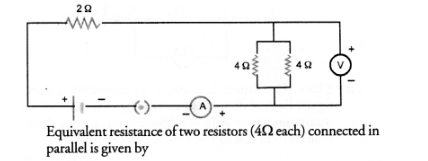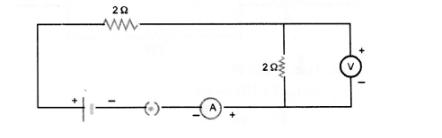# Draw a circuit diagram of an electric circuit containing a cell,

Question:

Draw a circuit diagram of an electric circuit containing a cell, a key, an ammeter, a reistor of 2Ω in series with a combination of two resistors (4Ω each) in

parallel and a voltmeter across the energy. 1 kWh = 1000 Watt x 3600 s parallel combination. Will the potentional difference across the 2Ω resistor be

the same as that across the parallel combination of 4Ω resistors ? Give reason.

Solution:$\frac{1}{R}=\frac{1}{4}+\frac{1}{4}=\frac{1}{2}$ or $R=2 \Omega$

Therefore, the above circuit can be redrawn as given belowTherefore, potential difference across 2Ω resistor will be same ; as that of across the parallel combination of 4Ω resistors. V = IR.

As R and I in both the cases is same so V = same.